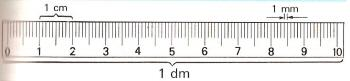Units of length to printName: ______________________________________  Subject: _______________________ Date: _______

Write on the right side what is missing.

1. The meter

We use the meter to measure the length and width of a classroom. The main unit of length is the meter that is defined as the distance between two lines on a platinum-iridium bar, preserved at the International Bureau of Weights and Measures in Paris.

The abbreviation for meters is m.2. Multiples of the meter

To express long distances as a race through the park, we use greater units than the meter. These are called multiples:

1 decameter is equal to 10 meters: 1 dam = 10 m.
1 hectometer is equal to 100 meters: 1 hm = 100 m.
1 kilometer is equal to 1000 meters: 1 km = 1000 m.
1 myriameter is equal to 10000 meters: 1 mym = 10000 m.

 3 hm = 8 dam = 7 km = 5 mam = 2 dam = 1 hm = 4 km = 6 mam =3. - Submultiples of the meter

To express small distances as the length and width of a sheet of paper, we use smaller units than the meter. These are called submultiples:

1 decimeter is equal to 0.1 meters: 1 dm = 0.1 m. 1 meter is equal to 10 decimeters.
1 centimeter is equal to 0.01 meters: 1 cm = 0.01 m. 1 meter is equal to 100 centimeters.
1 millimeter is equal to 0.001 meters: 1 mm = 0.001 m. 1 meter is equal to 1000 millimeters.

 3 cm = 5 dm = 2 mm = 4 dm = 7 mm = 6 cm =4. Converting from one unit to another

Each unit of length is 10 times greater than the immediate lower, and 10 times less than the immediate superior.

To convert from hm to dam, we multiply or move the decimal point one place to the right.

Examples: 7 hm = 70 dam = 700 m; 3 km = 30 hm = 300 dam = 3000 m.
7.35 m = 73. 5 dm = 735 cm = 7350 mm.

 7,28 km = 8 hm = 6,3 dam = 5,12 mam = 3,2 m = 83 cm =

5. Converting from one unit to another superior

To convert from m to dam, we divide the number by 10 or we move the decimal point one place to the left.

Examples: 70 m = 7 dam; 325 m = 32,5 dam = 3,25 hm = 0,325 km = 0,0325 mam.

 637 cm = 38 mm = 471 m = 1243 dam = 25 hm =

6. Problems

Do these problems on a paper and write the solution:

 1. Roberto takes a stroll in a bike and goes over 4.2 km. How many m did he go over? 2. A piece of cloth is 3 dam and 7 m and we have sold 2 dam and 3 m. How many dm of cloth do we still have to sell? 3. We have a table that is 65 dm long and we cut a piece of 257 cm. How many cm do we have? 4. A Street is 450 m long. How many m do we have to add to the street is 1 km long? 5. A guy wants to go over 7 km. If he walked 2345 m, how many m does he still have to go over to finish his walk?

| Educational applications | Mathematics |
In Spanish  | Interactive

«Arturo Ramo GarcĒa.-Record of intellectual property of Teruel (Spain) No 141, of 29-IX-1999
Plaza Playa de Aro, 3, 1║ DO 44002-TERUEL